# `ifermi` program¶

IFermi includes command-line tools for generating, analysing, and plotting Fermi surfaces. The tools can be accessed using the `ifermi` command-line program. All of the options provided in the command-line are also accessible using the Python API.

IFermi works in 4 stages:

1. It loads a band structure from DFT calculation outputs.

2. It interpolates the band structure onto a dense k-point mesh using Fourier interpolation as implemented in BoltzTraP2.

3. It extracts the Fermi surface at a given energy level.

4. It extracts information about the Fermi surface or plots it using several plotting backends.

Note

Currently, IFermi’s command-line tools only work with VASP calculation outputs. Support for additional DFT packages will be added in a future release.

IFermi is controlled on the command-line using the `ifermi` command. The available options can be listed using:

```ifermi -h
```

Information on the Fermi surface area, dimensionality, and orientation can be extracted using the `info` subcommand. The only input required is a vasprun.xml. For example:

```ifermi info
```

An example output for MgB2 is shown below:

```Fermi Surface Summary
=====================

# surfaces: 5
Area: 32.745 Å⁻²

Isosurfaces
~~~~~~~~~~~

Band    Area [Å⁻²]   Dimensionality    Orientation
------  ------------  ----------------  -------------
6         1.944         2D           (0, 0, 1)
7         4.370         1D           (0, 0, 1)
7         2.961         2D           (0, 0, 1)
8         3.549         1D           (0, 0, 1)
8         3.549         1D           (0, 0, 1)
```

If properties are included in the Fermi surface (see Property projections), the averaged property values will also be calculated. This allows for calculation of the Fermi velocity. For example:

```ifermi info --property velocity
```
```Fermi Surface Summary
=====================

# surfaces: 5
Area: 32.75 Å⁻²
Avg velocity: 9.131e+05 m/s

Isosurfaces
~~~~~~~~~~~

Band    Area [Å⁻²]    Velocity avg [m/s]   Dimensionality    Orientation
------  ------------  --------------------  ----------------  -------------
6         1.944             7.178e+05         2D           (0, 0, 1)
7         4.370             9.092e+05      quasi-2D        (0, 0, 1)
7         2.961             5.880e+05         2D           (0, 0, 1)
8         3.549             1.105e+06      quasi-2D        (0, 0, 1)
8         3.549             1.105e+06      quasi-2D        (0, 0, 1)
```

Fermi surfaces and slices can be visualised using the `plot` subcommand. Again, the only input required is a vasprun.xml file. For example:

```ifermi plot
```

The two subcommands `info` and `plot` share many of the same options which we describe below.

## Generation options¶

There are several options affect the generation of Fermi surfaces from ab initio calculation outputs. These options are available for both the `info` and `plot` subcommands.

### Input file¶

IFermi will look for a vasprun.xml or vasprun.xml.gz file in the current directory. To specify a particular vasprun file the `--filename` (or `-f` for short) option can be used:

```ifermi plot --filename my_vasprun.xml
```

### Interpolation factor¶

The band structure extracted from the vasprun must be processed before the Fermi surface can be generated. The two key issues are:

1. It may only contain the irreducible portion of the Brillouin zone (if symmetry was used in the calculation) and therefore may not contain enough information to plot the Fermi surface across the full reciprocal lattice.

2. It may have been calculated on a relatively coarse k-point mesh and will therefore produce a rather jagged Fermi surface.

Both issues can be solved by interpolating the band structure onto a denser k-point mesh. This is achieved by using BoltzTraP2 to Fourier interpolate the eigenvalues onto a denser mesh that covers the full Brillouin zone.

The degree of interpolation is controlled by the `--interpolation-factor` (`-i`) argument. A value of 8 (the default value), roughly indicates that the interpolated band structure will contain 8x as many k-points. Increasing the interpolation factor will result in smoother Fermi surfaces. For example:

```ifermi plot --interpolation-factor 10
```

Warning

As the interpolation increases, the generation of the Fermi surface, analysis and plotting will take a longer time and can result in large file sizes.

### Fermi surface energy¶

The energy level offset at which the Fermi surface is calculated is controlled by the `--mu` option. The energy level is given relative to the Fermi level of the VASP calculation and is given in eV. By default, the Fermi surface is calculated at `mu = 0`, i.e., at the Fermi level.

For gapped materials, `mu` must be selected so that it falls within the conduction or valence bands otherwise no Fermi surface will be obtained. For example. The following command will generate the Fermi surface at 1 eV above the Fermi level:

```ifermi plot --mu 1
```

### Property projections¶

Additional properties, such as the group velocity and orbital magnetisation (spin texture), can be projected onto the Fermi surface using the `--property` option. The group velocities are calculated during Fourier interpolation (units of m/s) and can be included in the Fermi surface using:

```ifermi plot --property velocity
```

For calculations performed using spin–orbit coupling or non-collinear magnetism, the spin magnetisation can be projected onto the Fermi surface using:

```ifermi plot --property spin
```

Warning

Projecting the spin magnetisation requires the k-point mesh to cover the entire Brillouin zone. I.e., the DFT calculation must have been performed without symmetry (`ISYM = - 1` in VASP).

It is possible to calculate the scalar projection of the the Fermi surface properties onto a cartesian axis using the `--projection-axis` option.. For example, to use the scalar projection of the spin magnetisation onto the [0 0 1] cartesian direction:

```ifermi plot --property spin --projection-axis 0 0 1
```

### Reciprocal space¶

By default, the Wigner–Seitz cell is used to contain to the Fermi surface. The parallelepiped reciprocal lattice cell can be used instead by selecting the `--no-wigner` option. For example:

```ifermi plot --no-wigner
```

## Visualisation options¶

In addition to the options for generating Fermi surfaces, there are several options that control the visualisation parameters. These options are only available for the `plot` subcommand.

### Plotting backend¶

IFermi supports multiple plotting backends. The default is to the plotly package but matplotlib and mayavi are also supported.

Note

The mayavi dependencies are not installed by default. To use this backend, follow the installation instructions here and then install IFermi using `pip install ifermi[mayavi]`.

Different plotting packages can be specified using the `--type` option (`-t`). For example, to use matplotlib:

```ifermi plot --type matplotlib
```

### Output files¶

By default, IFermi generates interactive plots. To generate static images, an output file can be specified using the `--output` (`-o`) option. For example:

```ifermi plot --output fermi-surface.jpg
```

Note

Saving graphical output files with the plotly backend requires plotly-orca to be installed.

Running the above command in the `examples/MgB2` directory produces the plot: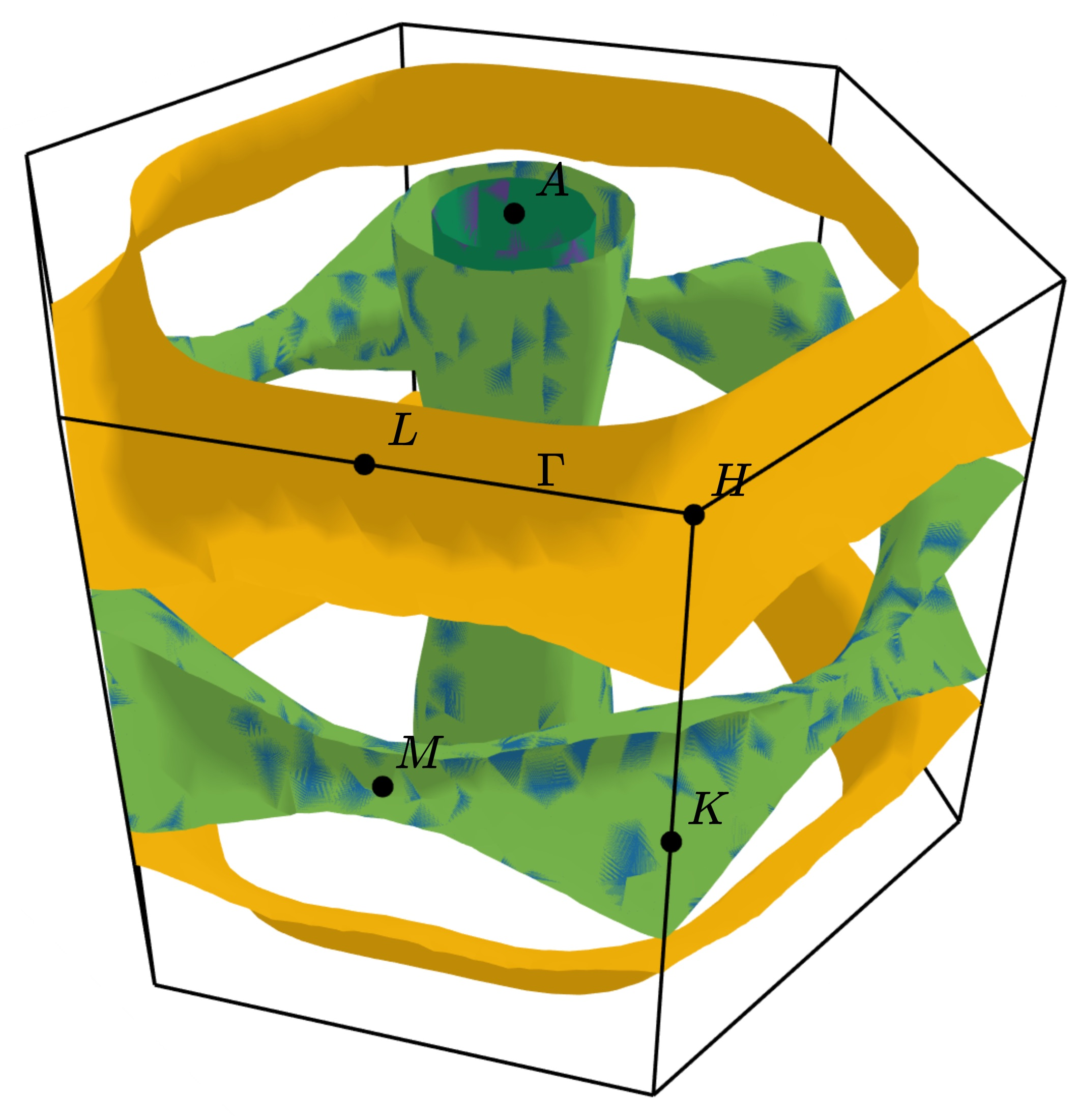Interactive plots can be saved to a html file using the plotly backend by specifying a html filename. This will prevent the plot from being opened automatically.

```ifermi plot --output fermi-surface.html
```

### Selecting spin channels¶

In the plot above, the spins are degenerate (the Hamiltonian does not differentiate between the up and down spins). This is why the surface looks dappled, IFermi is plotting two redundant surfaces. To stop it from doing this, we can specify that only one spin component should be plotted using the `--spin` option. The default is to plot both spins but a single spin channel can be selected through the names “up” and “down”. For example:

```ifermi plot --spin up
```### Changing the viewpoint¶

The viewpoint (camera angle) can be changed using the `--azimuth` (`-a`) and `--elevation` (`-e`) options. This will change both the initial viewpoint for interactive plots, and the final viewpoint for static plots. To summarize:

• The azimuth is the angle subtended by the viewpoint position vector on a sphere projected onto the x-y plane in degrees. The default is 45°.

• The elevation (or zenith) is the angle subtended by the viewpoint position vector and the z-axis. The default is 35°.

The viewpoint always directed to the center of the the Fermi surface (position [0 0 0]). As an example, the viewpoint could be changed using:

```ifermi plot --azimuth 120 --elevation 5
```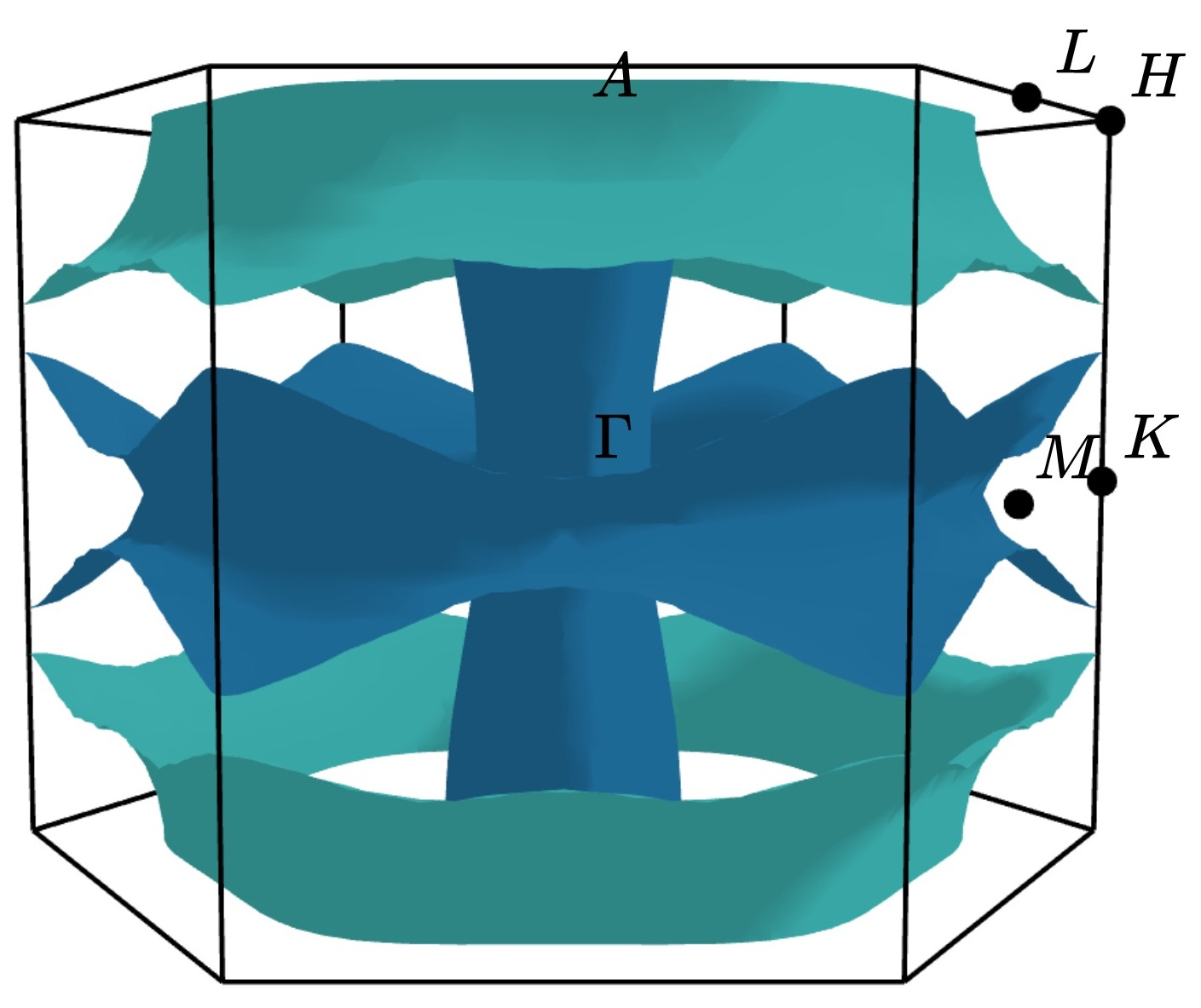### Styling face properties¶

As described in the Property projections section, Fermi surfaces (and Fermi slices) can include a property projected onto the isosurface faces. By default, if properties are included in the Fermi surface they will be indicated by coloring of the isosurface. If the face property is a vector, the norm of the vector will be used as the color intensity. The colormap of the surface can be changed using the `--property-colormap` option. All matplotlib colormaps are supported. For example:

```ifermi plot --property velocity --property-colormap viridis
```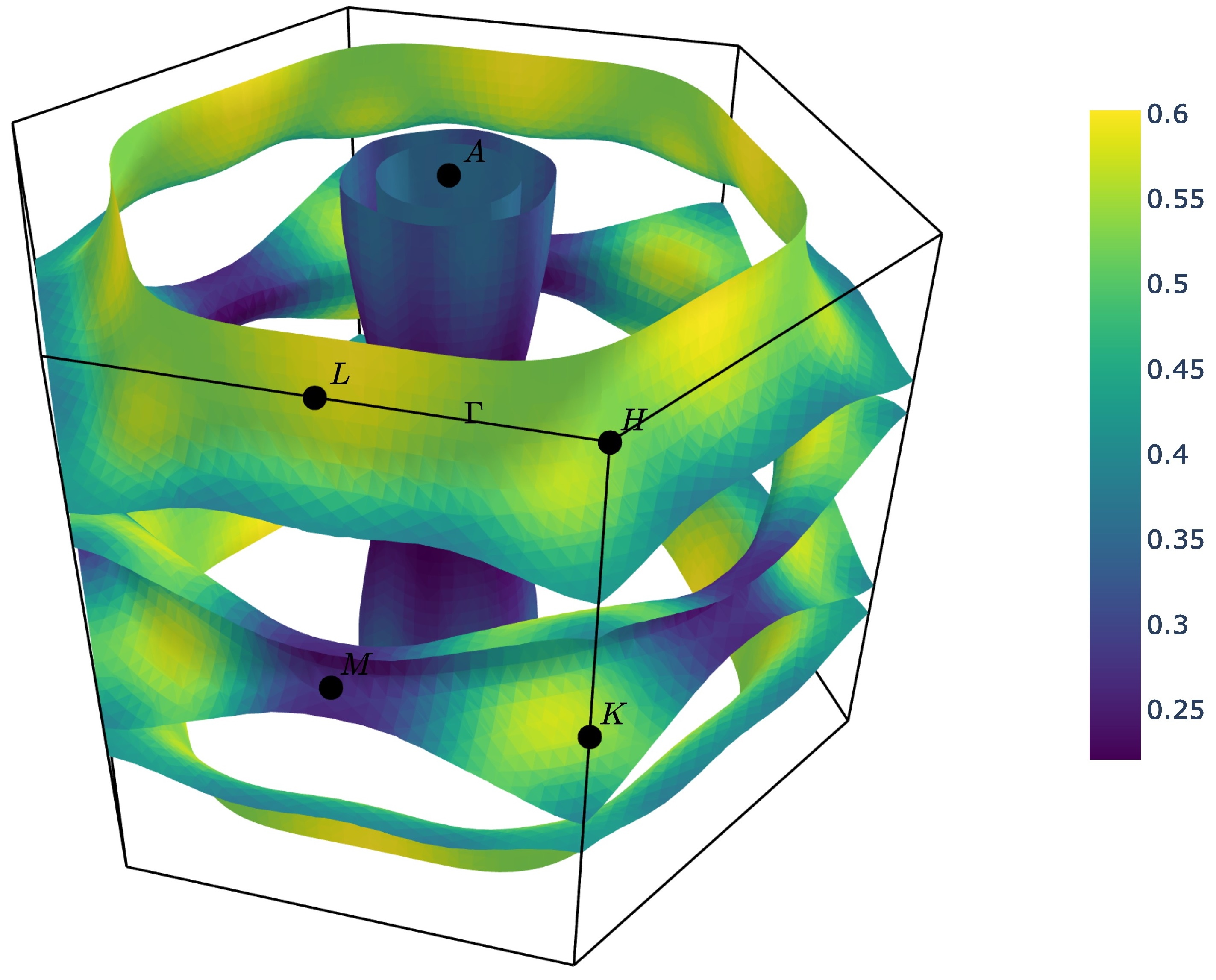The minimum and maximum values for the colorbar limits can be set using the `--cmin` and `--cmax` parameters. These should be used when quantitatively comparing surface properties between two plots. For example:

```ifermi plot --property velocity --cmin 0 --cmax 5
```

As described above, it is also possible calculate the scalar projection of the face properties onto a cartesian axis using the `--projection-axis` option. When combined with a diverging colormap this can be used to indicate surface properties that vary between positive and negative numbers. For example, below we color the Fermi surface of MgB2 by the projection of the group velocity onto the [0 0 1] vector (z-axis).

```ifermi plot --property velocity --projection-axis 0 0 1 --property-colormap RdBu
```Vector valued Fermi surface properties (such as group velocity or spin magnetisation) can also be visualised as arrows using the `--vector-property` option. If `--projection-axis` is set, the color of the arrows will be determined by the scalar projection of the property vectors onto the specified axis, otherwise the norm of the projections will be used. The colormap used to color the arrows is specified using `--vector-colormap`. Lastly, often it is useful to hide the isosurface (`--hide-surface` option) or high-symmetry labels (`-hide-labels`) when visualising arrows. An example of how to combine these options is given below:

```ifermi plot --property velocity --projection-axis 0 0 1 --property-colormap RdBu \
--vector-property --vector-colormap RdBu --hide-surface --hide-labels
```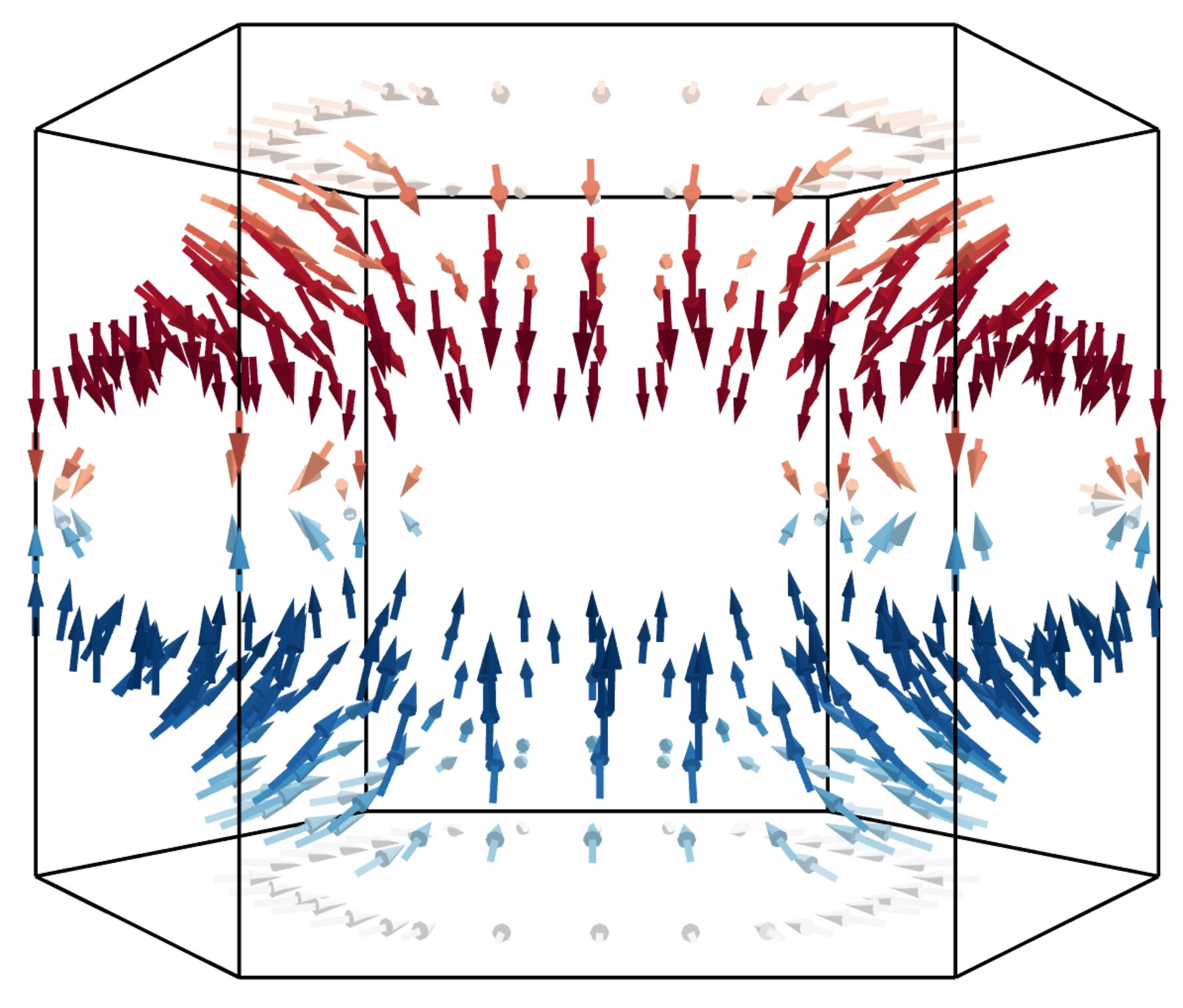The size of the arrows can be controlled using the `--vnorm` parameter. This is particularly useful when quantitatively comparing vector properties across multiple Fermi surfaces. A larger `vnorm` value will increase the size of the arrows. The spacing between the arrows is controlled by the `--vector-spacing` option. Smaller values will increase the density of the arrows.

### Fermi slices¶

IFermi can also generate two-dimensional slices of the Fermi surface along a specified plane using the `--slice` option. Planes are defined by their miller indices (a b c) and a distance from the plane, d. Most of the above options also apply to to Fermi slices, however, slices are always plotted using matplotlib as the backend.

For example, a slice through the (0 0 1) plane that passes through the center of the Brillouin zone (Γ-point) can be generated using:

```ifermi plot --slice 0 0 1 0
```Slices can contain segment properties in the same way that surfaces can contain face properties. To style slices with projections see Styling face properties. When including arrows in Fermi slice figures, only the components of the arrows in the 2D plane will be shown. As an example below we plot the spin texture of BiSb (`examples/BiSb`) with and without arrows. The spin texture is colored by the projection of the spin onto the [0 0 1] cartesian direction.

Without arrows:

```ifermi plot --mu -0.85  -i 10 --slice 0 0 1 0 --property spin --hide-cell \
--hide-labels --projection-axis 0 1 0 --property-colormap RdBu
```With arrows:

```ifermi plot --mu -0.85  -i 10 --slice 0 0 1 0 --property spin --hide-cell \
--hide-labels --projection-axis 0 1 0 --property-colormap RdBu \
--vector-property --vector-colormap RdBu --vnorm 5 --vector-spacing 0.025
```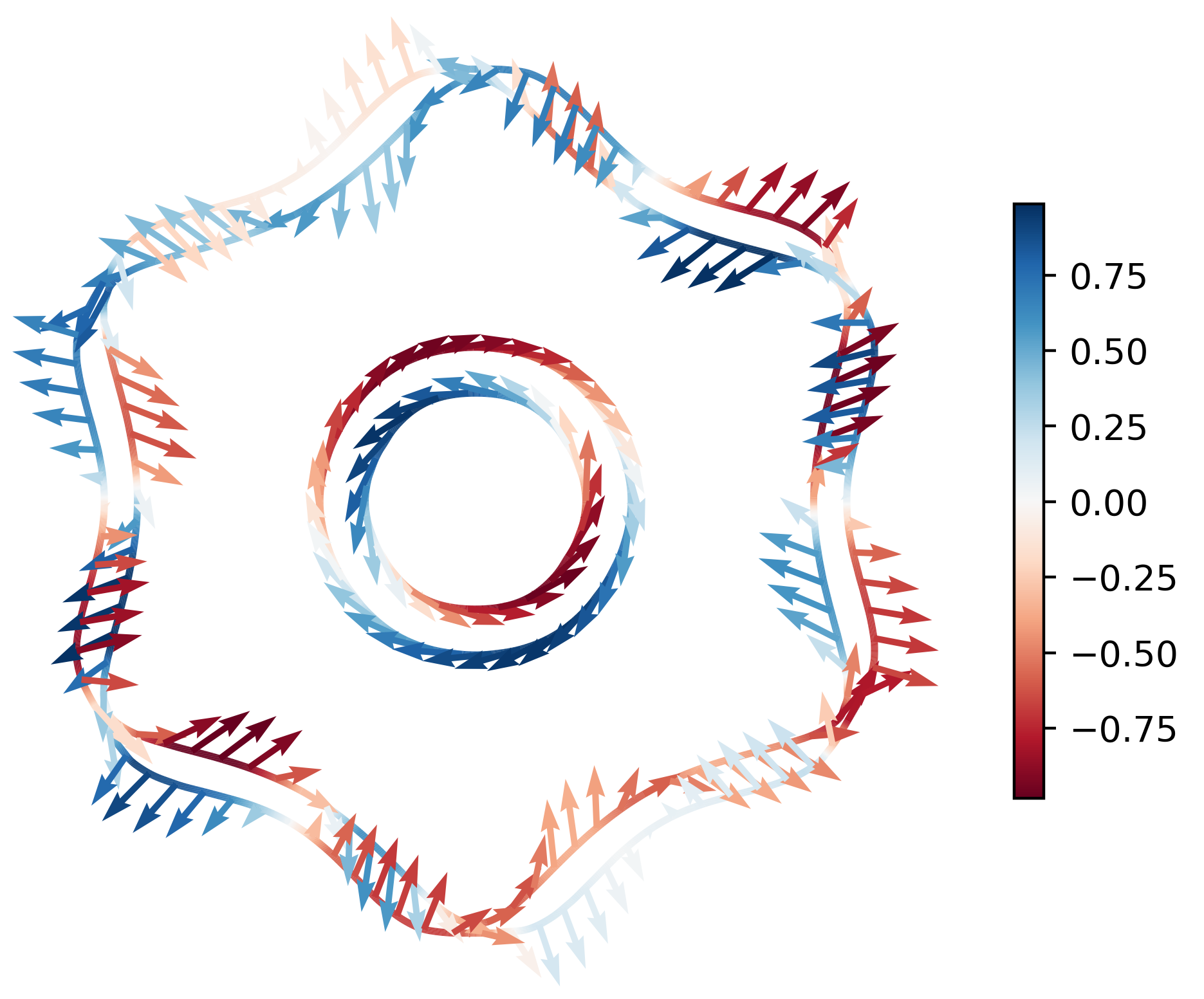Warning

When generating spin texture plots for small regions of k-space, for example, in a small area around the Γ-point, it is often necessary to increase the k-point mesh density of the underlying DFT calculation. In the example above, the DFT calculation was performed on a 21x21x21 k-point mesh.

Furthermore, projecting the spin magnetisation requires the k-point mesh to cover the entire Brillouin zone. I.e., the DFT calculation must have been performed without symmetry (`ISYM = - 1` in VASP).

## `ifermi` reference¶

### ifermi¶

IFermi is a tool for the generation, analysis and plotting of Fermi surfaces.

```ifermi [OPTIONS] COMMAND [ARGS]...
```

#### info¶

Calculate information about the Fermi surface.

```ifermi info [OPTIONS]
```

Options

-f, --filename <filename>

vasprun.xml file to plot

-m, --mu <mu>

offset from the Fermi level at which to calculate Fermi surface

Default

0.0

--wigner, --no-wigner

use Wigner-Seitz cell rather than reciprocal lattice parallelepiped

Default

True

-i, --interpolation-factor <interpolation_factor>

interpolation factor for band structure

Default

8.0

--property <property>

projection type

Options

velocity | spin

--projection-axis <projection_axis>

use dot product of property onto cartesian axis (e.g. 0 0 1)

--decimate-factor <decimate_factor>

factor by which to decimate surfaces (i.e. 0.8 gives 20 % fewer faces)

--smooth

smooth the Fermi surface

Default

False

--norm, --no-norm

average property norms (overridden by –projection-axis)

Default

True

--precision <precision>

number of decimal places in output

Default

4

#### plot¶

Plot a Fermi surface from a vasprun.xml file.

```ifermi plot [OPTIONS]
```

Options

-f, --filename <filename>

vasprun.xml file to plot

-m, --mu <mu>

offset from the Fermi level at which to calculate Fermi surface

Default

0.0

--wigner, --no-wigner

use Wigner-Seitz cell rather than reciprocal lattice parallelepiped

Default

True

-i, --interpolation-factor <interpolation_factor>

interpolation factor for band structure

Default

8.0

--property <property>

projection type

Options

velocity | spin

--projection-axis <projection_axis>

use dot product of property onto cartesian axis (e.g. 0 0 1)

--decimate-factor <decimate_factor>

factor by which to decimate surfaces (i.e. 0.8 gives 20 % fewer faces)

--smooth

smooth the Fermi surface

Default

False

-o, --output <output_filename>

output filename

-s, --symprec <symprec>

symmetry precision in Å

Default

0.001

-a, --azimuth <azimuth>

viewpoint azimuth angle in °

Default

45.0

-e, --elevation <elevation>

viewpoint elevation angle in °

Default

35.0

-t, --type <plot_type>

plotting type

Default

plotly

Options

matplotlib | plotly | mayavi

--color-property, --no-color-property

color Fermi surface properties

Default

True

--property-colormap <property_colormap>

matplotlib colormap name for properties

--vector-property, --no-vector-property

show vector properties as arrows

Default

False

--vector-colormap <vector_colormap>

matplotlib colormap name for vectors

--vector-spacing <vector_spacing>

spacing between property arrows

Default

0.2

--cmin <cmin>

minimum intensity on property colorbar

--cmax <cmax>

maximum intensity on property colorbar

--vnorm <vnorm>

value by which to normalise vector lengths

--scale-linewidth

scale Fermi slice thickness by property

Default

False

--hide-surface

hide the Fermi surface

Default

False

--plot-index <plot_index>

plot specific bands indices (1 based)

--hide-labels

hide the high-symmetry k-point labels

Default

False

--hide-cell

hide reciprocal cell boundary

Default

False

--spin <spin>

select spin channel

Options

up | down

--slice <slice>

slice through the Brillouin zone (format: j k l dist)

--scale <scale>

scale for image resolution

Default

4